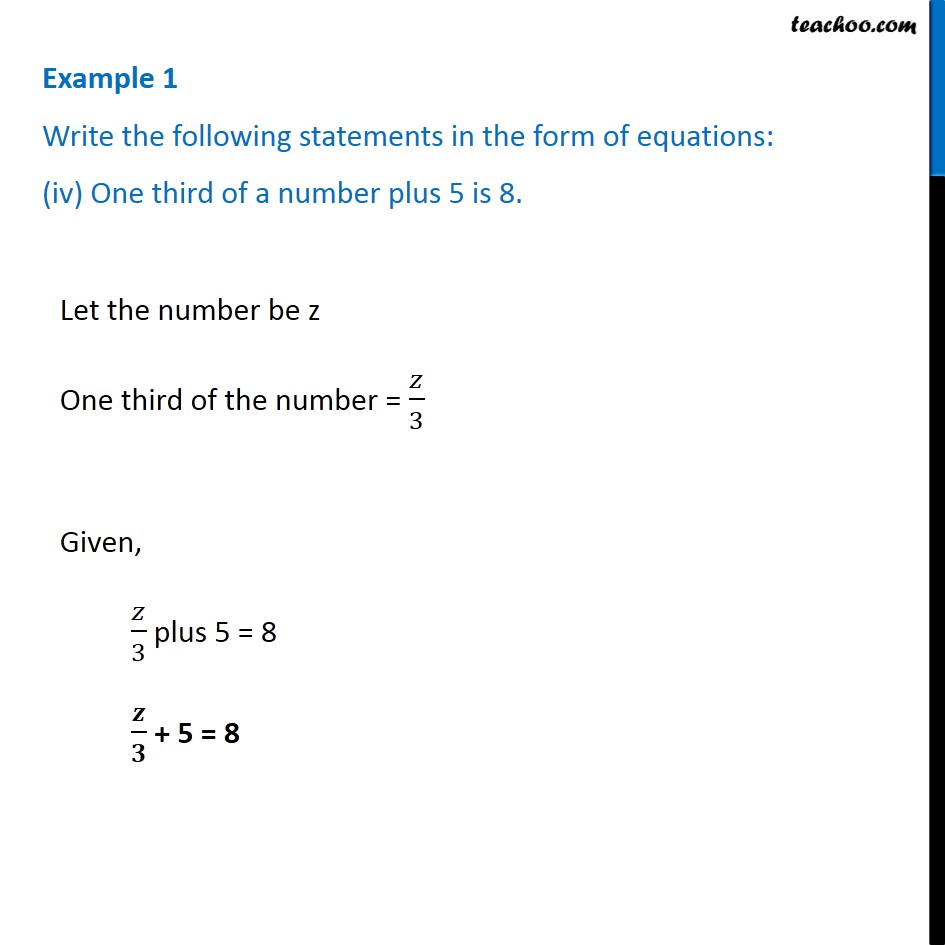Examples

Chapter 4 Class 7 Simple Equations
Serial order wiseLearn in your speed, with individual attention - Teachoo Maths 1-on-1 Class

### Transcript

Example 1 Write the following statements in the form of equations: (iv) One third of a number plus 5 is 8. Let the number be z One third of the number = 𝑧/3 Given, 𝑧/3 plus 5 = 8 𝒛/𝟑 + 5 = 8